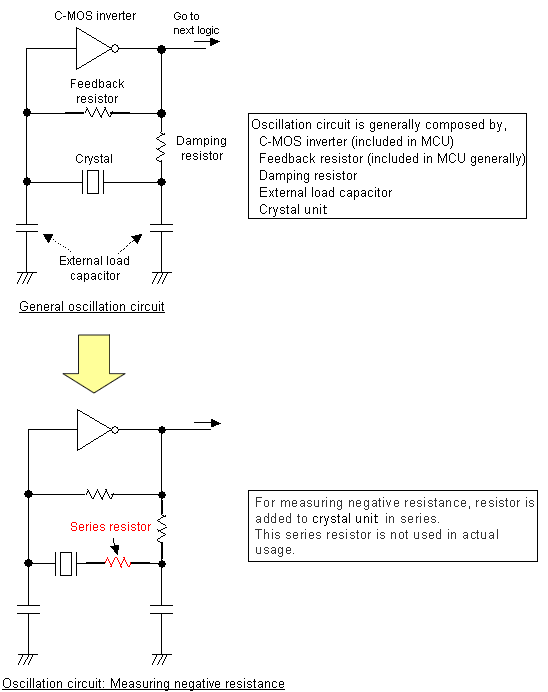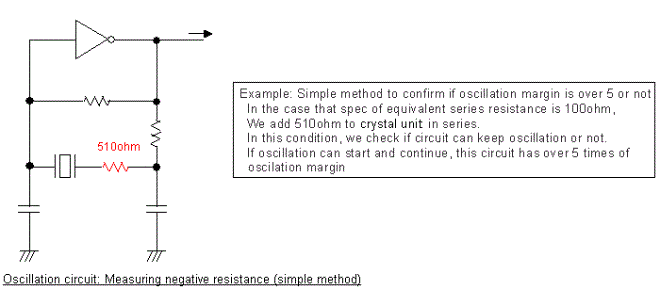# Crystal UnitsOscillation margin

Basic Knowledge of Crystal Units

No. Item Title Contents
1 Outline Oscillation margin

It is a margin to the oscillation stop and the most important item in the oscillation circuit.
This margin is indicated by ratio based on resistance of crystal, and it shows how amplification capability oscillation circuit has.

The oscillation circuit can theoretically operate if oscillation margin is 1 or more. However, if oscillation margin is close to 1, it is possible that operation failure will occur on module by excessive long oscillation start up time and so on. Such problems will be able to be solved by larger oscillation margin.

2 Measurement Calculation Oscillation margin is able to be calculated as follows.
Oscillation margin [times] = |-R| / R1spec
|-R|: Negative Resistance
R1spec: Maximum value of equivalent series resistance of crystal unit specified in specification
Please refer catalog or datasheet of our crystal for R1 spec.
It is possible to measure Negative Resistance on the actual oscillation circuit.
It is better to keep 5 times or more as oscillation margin.
Measuring method
1. Requirements for measurement
• PCB
• Crystal unit (with equivalent circuit constants data)
• Resistors (SMD)
• Measurement equipment (Oscilloscope, Frequency counter or others capable to observe oscillation)
2. Add resistor to resonator in serial and check if oscillation circuit works or not.
3.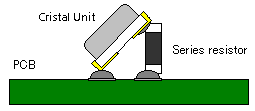4. If oscillation is confirmed by 2), change resistance large. If there is no oscillation, change resistance small.
5. Find out maximum resistance (=Rs_max) which is resistace just before the oscillation stop.
6. Measure oscillating frequency with Rs_max.
7. Calculate effective resistance; RL by formula below.
8.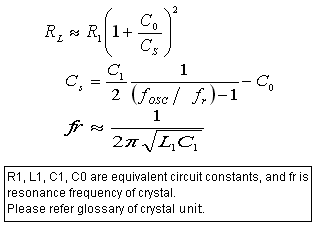9. Calculate negative resistance; |-R| by formula below.
10.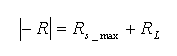Simple method
• We show simple method to check if oscillation margin is over 5 times or not.
1. Prepare resistor which is 5 times resistance to spec of equivalent series resistance of crystal unit.
2. Add prepared resistor to crystal unit in series.
3. Check if oscillation circuit works normally.
• Judgement
If oscillation circuit works normally, it means that margin for oscillation stop is 5 times or more.
If oscillation circuit does not work, margin for oscillation stop might be less than 5 times.
If margin for oscillation stop is less than 5, it is better to change damping resistance or external load capacitance smaller. (Cause and measures for oscillation stop)
3 Judgement   Please use oscillation circuit with over 5 times oscillation margin.
In actual usage, variation of oscillation margin should be considered though oscillation circuit can work if oscillation margin is 1 or more, theoretically.
Possibility of oscillation trouble might be high with low oscillation margin. Therefore, it is better to check oscillation margin and consider circuit conditions to keep enough oscillation margin.
4 Notes
• Oscillation margin is affected not only by crystal characterisitcs but also parts that compose oscillation circuit (MCU, capacitor and resistor). Therefore, it is better to check oscillation margin when you fix using MCU on your module.
• The series resistor is only for the evaluation. Please do not use this resistor in actual usage.
• It is better to check function of your module also. It is possible that module does not work correctly by frequency shift on oscillation circuit and so on.
• Jig and socket could be used in measurement, but stray of them will give influence for oscillation margin.